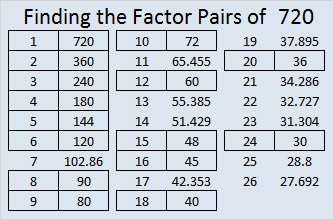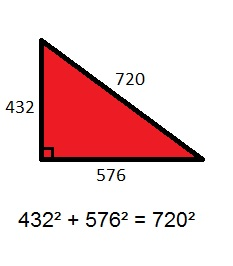# 720 Christmas Factor Trees

720 has 30 factors. That’s more than any previous number!

• 720 is a composite number.
• Prime factorization: 720 = 2 x 2 x 2 x 2 x 3 x 3 x 5, which can be written 720 = (2^4) x (3^2) x 5
• The exponents in the prime factorization are 4, 2 and 1. Adding one to each and multiplying we get (4 + 1)(2 + 1)(1 + 1) = 5 x 3 x 2 = 30. Therefore 720 has exactly 30 factors.
• Factors of 720: 1, 2, 3, 4, 5, 6, 8, 9, 10, 12, 15, 16, 18, 20, 24, 30, 36, 40, 45, 48, 60, 72, 80, 90, 120, 144, 180, 240, 360, 720
• Factor pairs: 720 = 1 x 720, 2 x 360, 3 x 240, 4 x 180, 5 x 144, 6 x 120, 8 x 90, 9 x 80, 10 x 72, 12 x 60, 15 x 48, 16 x 45, 18 x 40, 20 x 36 or 24 x 30
• Taking the factor pair with the largest square number factor, we get √720 = (√144)(√5) = 12√5 ≈ 26.8328157.6 ⋅ 5 ⋅ 4 ⋅ 3 ⋅ 2 ⋅ 1 = 720, and is usually written 6! = 720.

Since I hosted a Christmas Eve dinner for my extended family, I thought of some of the many ways people could fill up their plates.If we made a tree diagram of all the possible dinners that could be made with exactly one item from each column, it would contain 720 lines and require quite a few pages.

The fundamental counting principle tells us the easiest way to count all those dinners is to multiply together the number of items in each column. In this case that would be 6 ⋅ 5 ⋅ 4 ⋅ 3 ⋅ 2 ⋅ 1 = 720.

On the other hand, factor trees for 720 are easy to make.

Here are some of MANY possible factor trees for 720:None of those were very attractive, but here are some that are much better looking:Here are some other random facts about the number 720:

Because 5 is one of its factors, 720 is the hypotenuse of the Pythagorean triple 432-576-720. What is the greatest common factor of those 3 numbers? The greatest common factor is in the factor pair with the number 5. All 15 factor pairs for 720 are listed at the end of the post.The sum of the interior angles of any hexagon is 720 degrees.720 is a palindrome in three different bases:

• 5A5 in base 11; note that 5(121) + 10(11) + 5(1) = 720.
• OO in base 29; (O is 24 base 10) note that 24(29) + 24(1) = 720.
• KK in base 35; (K is 20 base 10) note that 20(35) + 20(1) = 720.

———————————————————